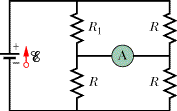# Problem: In the figure R1 = 2.75R, the ammeter resistance is zero, and the battery is ideal. What multiple of ε/R gives the current in the ammeter?

###### FREE Expert Solution

Let the current through resistor R be i.

From the figure, the voltage across the ammeter is zero making the current in the bottom resistors to be the same.

The current through the battery is then 2i.

The voltage drop across the bottom resistors, VR = iR.

Equivalent resistance for 2 resistors in parallel:

$\overline{){{\mathbf{R}}}_{{\mathbf{eq}}}{\mathbf{=}}\frac{{\mathbf{R}}_{\mathbf{1}}{\mathbf{R}}_{\mathbf{2}}}{{\mathbf{R}}_{\mathbf{1}}\mathbf{+}{\mathbf{R}}_{\mathbf{2}}}}$

Equivalent resistance for resistors in series:

$\overline{){{\mathbf{R}}}_{{\mathbf{eq}}}{\mathbf{=}}{{\mathbf{R}}}_{{\mathbf{1}}}{\mathbf{+}}{{\mathbf{R}}}_{{\mathbf{2}}}{\mathbf{+}}{\mathbf{.}}{\mathbf{.}}{\mathbf{.}}{\mathbf{+}}{{\mathbf{R}}}_{{\mathbf{n}}}}$

From these equations, the equivalent resistance of the circuit is:

$\begin{array}{rcl}{\mathbf{R}}_{\mathbf{e}\mathbf{q}}& \mathbf{=}& \mathbf{\left[}\frac{\mathbf{\left(}\mathbf{2}\mathbf{.}\mathbf{75}\mathbf{R}\mathbf{\right)}\mathbf{\left(}\mathbf{R}\mathbf{\right)}}{\mathbf{2}\mathbf{.}\mathbf{75}\mathbf{R}\mathbf{+}\mathbf{R}}\mathbf{\right]}\mathbf{+}\mathbf{\left[}\frac{\mathbf{\left(}\mathbf{R}\mathbf{\right)}\mathbf{\left(}\mathbf{R}\mathbf{\right)}}{\mathbf{R}\mathbf{+}\mathbf{R}}\mathbf{\right]}\\ & \mathbf{=}& \frac{\mathbf{2}\mathbf{.}\mathbf{75}{\mathbf{R}}^{\mathbf{2}}}{\mathbf{3}\mathbf{.}\mathbf{75}\mathbf{R}}\mathbf{+}\frac{{\mathbf{R}}^{\mathbf{2}}}{\mathbf{2}\mathbf{R}}\\ & \mathbf{=}& \frac{\mathbf{2}\mathbf{.}\mathbf{75}\mathbf{R}}{\mathbf{3}\mathbf{.}\mathbf{75}}\mathbf{+}\frac{\mathbf{R}}{\mathbf{2}}\end{array}$

97% (477 ratings)###### Problem Details

In the figure R1 = 2.75R, the ammeter resistance is zero, and the battery is ideal. What multiple of ε/R gives the current in the ammeter?